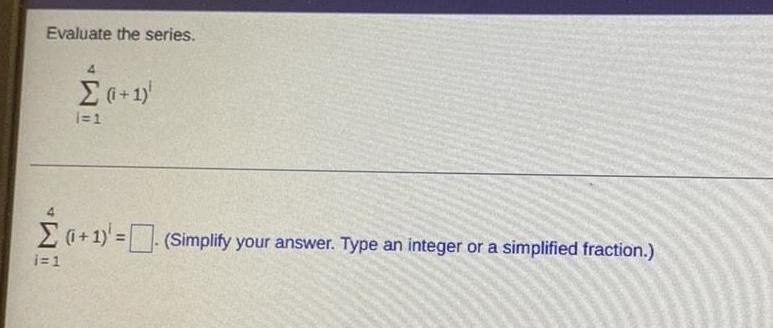Question:

# Evaluate the series. 4Σ (i+1) i=1 i=14Σ (i+1) = (Simplify

Last updated: 8/11/2022Evaluate the series. 4Σ (i+1) i=1 i=14Σ (i+1) = (Simplify your answer. Type an integer or a simplified fraction.)